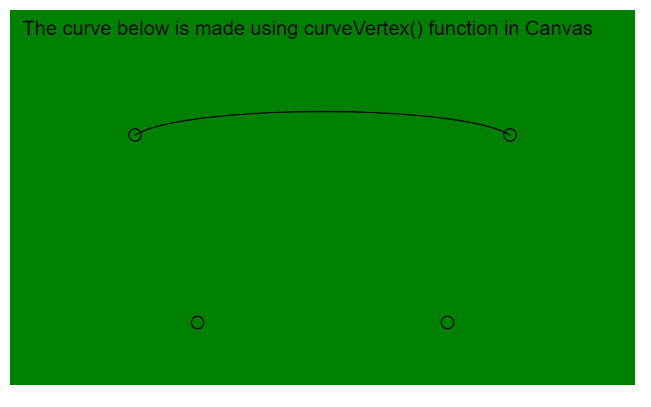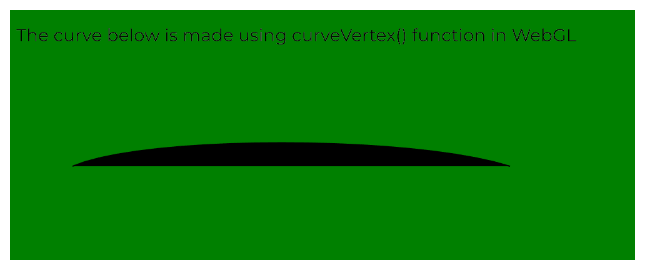# p5.js | curveVertex() Function

• Last Updated : 25 May, 2020

The curveVertex() function in p5.js is used to specify the vertex coordinates used to draw a curve. It expects 2 parameters for 2D curves and 3 parameters for 3D curves. Both the 2D and 3D modes can be used for drawing in the WebGL mode. This function can only be used between the beginShape() and endShape().

The first and last vertices are used to guide the beginning and end of a curve. A minimum of four points is required to draw a curve between the given second and third points. Additional vertices would be used to draw the curve between them.

Syntax:

`curveVertex( x, y )`

OR

`curveVertex( x, y, [z] )`

Parameters: This function accepts three parameters as mentioned above and described below:

• x: It is a number that specifies the x-coordinate of the vertex.
• y: It is a number that specifies the y-coordinate of the vertex.
• z: It is a number that specifies the z-coordinate of the vertex. It is an optional parameter.

The examples below illustrates the curveVertex() function in p5.js:

Example 1:

 `function` `setup() {``  ``createCanvas(500, 300);``  ``textSize(16);``}`` ` `function` `draw() {``  ``background(``"green"``);``  ``fill(``"black"``);``  ``text(``"The curve below is made using curveVertex() function in Canvas"``, 10, 20);`` ` `  ``// Define the vertex points``  ``let p1 = { x: 150, y: 250 };``  ``let p2 = { x: 100, y: 100 };``  ``let p3 = { x: 400, y: 100 };``  ``let p4 = { x: 350, y: 250 };`` ` `  ``noFill();`` ` `  ``// Start the curve``  ``beginShape();`` ` `  ``// Specify other points in curveVertex()``  ``curveVertex(p1.x, p1.y);``  ``curveVertex(p2.x, p2.y);``  ``curveVertex(p3.x, p3.y);``  ``curveVertex(p4.x, p4.y);``  ``endShape();`` ` `  ``// Draw circles for demonstration``  ``circle(p1.x, p1.y, 10);``  ``circle(p2.x, p2.y, 10);``  ``circle(p3.x, p3.y, 10);``  ``circle(p4.x, p4.y, 10);``}`

Output:Example 2:

 `let newFont;`` ` `function` `preload() {``  ``newFont = loadFont(``"fonts/Montserrat.otf"``);``}`` ` `function` `setup() {``  ``createCanvas(500, 200, WEBGL);``  ``textFont(newFont, 14);``}`` ` `function` `draw() {``  ``background(``"green"``);``  ``fill(``"black"``);``  ``text(``"The curve below is made using curveVertex() function in WebGL"``, -245, -75);`` ` `  ``// Define the vertex points``  ``let p1 = { x: -200, y: 175, z: 0 };``  ``let p2 = { x: -200, y: 25, z: 0 };``  ``let p3 = { x: 150, y: 25, z: 0 };``  ``let p4 = { x: 275, y: 175, z: 0 };`` ` `  ``noFill();`` ` `  ``// Start the curve``  ``beginShape();`` ` `  ``// Specify the points of the vertex``  ``curveVertex(p1.x, p1.y, p1.z);``  ``curveVertex(p2.x, p2.y, p2.z);``  ``curveVertex(p3.x, p3.y, p3.z);``  ``curveVertex(p4.x, p4.y, p4.z);``  ``endShape();``}`

Output:Online editor: https://editor.p5js.org/

My Personal Notes arrow_drop_up